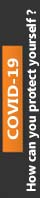Oct 16, 2017 term paper 2

# SAM Projects Office 2010

This paper concentrates on the primary theme of SAM Projects Office 2010 in which you have to explain and evaluate its intricate aspects in detail. In addition to this, this paper has been reviewed and purchased by most of the students hence; it has been rated 4.8 points on the scale of 5 points. Besides, the price of this paper starts from £ 40. For more details and full access to the paper, please refer to the site.

1.The Test Score worksheet contains a table of individual student scores and a table for the grading curve. In the Test Score worksheet, the range F4:G8 will contain the lower and upper ranges for each letter grade. Add the missing upper range values in the range G4:G7 by inserting formulas in each of those cells that calculates the upper range for each letter grade as being one point lower than the lower range of the next letter grade. Any changes to the numeric values in F5:F8 should result in changes to the calculated values in G4:G7.

2.In cell D4, enter the VLOOKUP function to return the letter grade for the first student in the list. The lookup value is the student’s final score, the table array is the cell range \$F\$4:\$H\$8, the column index number is 3, and the lookup should find the closest match in the first column of the lookup table. Copy the formula in cell D4 into the range D5:D223 to calculate the grades for the rest of the students’ scores.

3.In cell I4, use the COUNTIF function to count the total number of letter grades in the range \$D\$4:\$D\$223 equal to the value in cell H4 (e.g. “F”). Copy your formula into the range I5:I8 to count the total number of the other letter grades assigned under the current grading scale. In cell I9, calculate the total number of all letter grades, verifying that the total equals 220.

4.In the range J4:J8, calculate the percent of each letter grade assigned to the student body. In cell J9, calculate the total percentage of all letter grades, verifying that the total percentage equals 100 percent.

5.In the range L4:L8, use the ABS function to calculate the absolute value of the difference between the observed percentage of each letter grade and Professor Reynolds’ optimal percentage. In cell L9, calculate the total value of these absolute differences.

6.Assign the following range names:

a)      LowF, LowD, LowC, LowB, and LowA for the values in the range F4:F8.

b)      HighF through HighA for the values in the range G4:G8.

c)      PercentF through PercentA for the values in the range J4:J8.

d)      DifferenceFromCurve to the value in cell L9.

7.Enter the values of the five grading curve scenarios named Grading Curve 1 through Grading Curve 5 shown in the chart below into your scenarios. Use the range F4:F8 as your changing cells.su_table]

 Scenario Name Low F Low D Low C Low B Low A Grading Curve 1 0 20 40 60 80 Grading Curve 2 0 30 50 70 90 Grading Curve 3 0 50 65 80 95 Grading Curve 4 0 40 60 75 85 Grading Curve 5 0 60 70 80 90

8.Create a scenario summary report evaluating the results from each of the five scenarios, displaying the values from the range J4:J8,L9 as your result cells.

(Note: The closeness of each grading curve to Professor Reynolds’ optimal grading curve is expressed in the value of cell L9. If there is perfect correspondence, the value of cell L9 would be zero.)

9.Create a Solver model to minimize the value in cell L9 by changing the values in the range F5:F8, subject to the constraint that all of the values in the range F5:F8 must be integers.  Save the Solver model, selecting cell L13 as the top cell holding the solver model data.

0% Plagiarism Guaranteed & Custom Written, Tailored to your instructions

International House, 12 Constance Street, London, United Kingdom,
E16 2DQ

## STILL NOT CONVINCED?

We've produced some samples of what you can expect from our Academic Writing Service - these are created by our writers to show you the kind of high-quality work you'll receive. Take a look for yourself!FLAT 25% OFF ON EVERY ORDER.Use "FLAT25" as your promo code during checkout